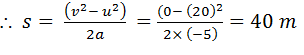# Consider a car moving along a straight horizontal road with a speed of 72 km/h. If the coefficient of static friction between the tyres and the road is 0.5, the shortest distance in which the car can be stopped is (taking g = 10 m/s2) (a)    30m (b)    40m         (c)    12m (d)    20m

9 years ago

(b)

Here u = 72 km/h = 20 m/s; v = 0;

a =- mg = -0.5 × 10 =-5m/s2

As v2 = u1 + 2as,